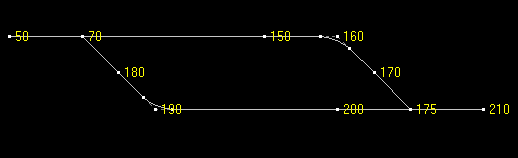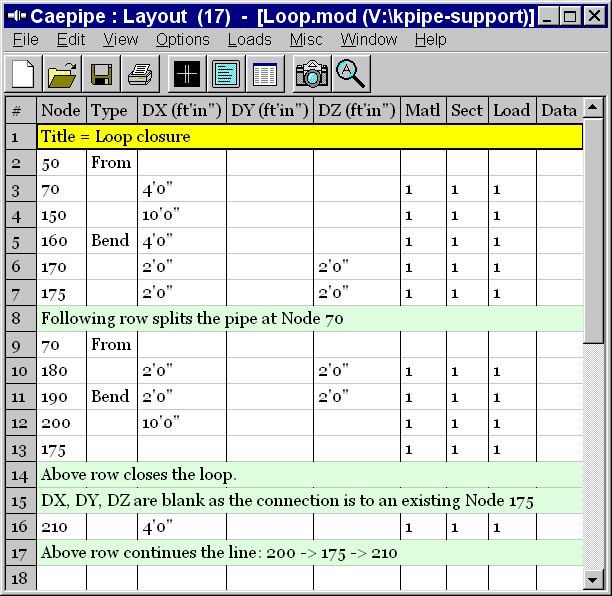## Tips January - March 2002

### How to Close a Loop in CAEPIPE

The following question comes up frequently: How do I close a loop?

It is simple in CAEPIPE. Please look at the example below. Note that CAEPIPE does not allow you to enter any offsets for a node that has been previously defined (node 175 in this example, row 13 in the second figure below).### Dynamic Analysis  Cut-off Frequency

Question: How do you estimate rigid-mode cut-off frequency?

Reply: The rigid-mode cut-off is sort of a traditional number or rule of thumb. When we started doing response spectrum analysis in the late 1960s, we used about 15 hz. (or 0.067 sec. period - I was a Civil Engineer then), it evolved to 20 hz. (or 0.05 sec. period), to 30 hz. in the 1970s (I was now a Mechanical Engineer), and some now use 60 hz. Personally, I don't think anything above 30 hz. is very meaningful. The 60 hz. is intended to cover high frequency building filtered shock-type loads in nuclear plants, but current nuclear facilities have very rigid structures and piping systems are very rigidly attached. I personally don't think the piping can respond very much and the stresses would then be relatively small.

Author: Mr. Ron Haupt, P. E., of Pressure Piping Engineering (www.ppea.net) is a member of several piping code committees (B31, B31.1, B31.3, BPTCS, and others). He consults with us in the capacity of Nuclear QA Manager.

### How to Use the Slope Command

The Slope feature was newly added in CAEPIPE v5.1. It is available under the Edit menu in the Layout screen.

The main function is to calculate automatically the offsets for a sloping element (that is not aligned along one of the major global axes). When a pipe slopes (or is routed at an angle) either in plane or out of it, it becomes necessary to calculate the offsets (DX, DY and DZ) using those faithful sine and cosine functions. But, therein lies the problem. Sometimes, it can get tricky and time-consuming.

But, not to worry. CAEPIPE's Slope feature will simplify the task. Let us see a few examples:

Example 1: A 20 degree line in the XZ plane, 10 feet long.

Method 1: Calculate the offsets DX = 10 cosine (20) = 9.4 (ft.), DZ = 10 sine (20) = 3.42 (ft.). Simple!

Method 2: Find direction cosines (DCs) for the line (vector). Direction cosines are simply cosines of the angles the vector makes with the global X, Y and Z axes. In this case, A=20° (X), B=90° (Y), C=70° (Z) deg. Cosines of these angles are: Xcomp=0.94, Ycomp=0, Zcomp=0.342.

Now, position the cursor on the sloping element row, right-click on DX/DY/DZ. Enter these numbers into the Xcomp, Ycomp and the Zcomp fields of the Slope dialog box that opens up and type the length, 10 ft. Press Enter. CAEPIPE will calculate the respective offsets using the DCs you input.

Once you have got the slope right, follow this pipe with other elements down the line by inputting different lengths while you retain the same direction cosines.

Example 2: A 40 degree line in the X(-Y) plane.

Method 2: Find direction cosines, if the line is at 40 deg. to X-axis in X(-Y) plane, it makes 50 deg. with the (-Y) axis. So, the DCs are: cos(40°) = 0.766, cos(50°) = 0.643, So, Xcomp = 0.766, Ycomp = 0.643, Zcomp = 0.0. Type these DCs and a length into the Slope dialog. Press Enter.